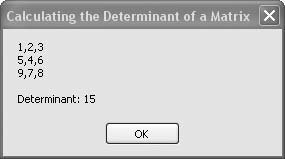# Recipe 6.33. Calculating the Determinant of a Matrix

#### Problem

You need to calculate the determinant of a matrix.

#### Solution

Sample code folder: Chapter 06\Matrix

#### Discussion

The determinant of a matrix is a single number derived from a matrix. It helps determine if a matrix is invertible, and it also comes into play when using matrices to solve simultaneous equations. (A full description of matrices and their operations is beyond the scope of this book.)

The following sample code creates a square matrix of double-precision numbers and passes it to the MatrixHelper.Determinant() function in the MatrixHelper module, which returns the determinant of the matrix:

` Dim matrixA(,) As Double = { _    {1, 2, 3}, _    {5, 4, 6}, _    {9, 7, 8}} Dim determinant As Double = MatrixHelper.Determinant(matrixA) MsgBox(MatrixHelper.MakeDisplayable(matrixA) & _    vbNewLine & vbNewLine & "Determinant: " & _    determinant.ToString) `

The complete MatrixHelper module is listed in Recipe 6.35. The Determinant() function is listed here for easy reference:

` Public Function Determinant(ByVal sourceMatrix(,) _       As Double) As Double    ' ----- Calculate the determinant of a matrix.    Dim result As Double    Dim pivots As Integer    Dim count As Integer    ' ----- Only calculate the determinants of square matrices.    If (UBound(sourceMatrix, 1) <> _          UBound(sourceMatrix, 2)) Then       Throw New Exception("Determinant only " & _          "calculated for square matrices.")    End If    Dim rank As Integer = UBound(sourceMatrix, 1)    ' ----- Make a copy of the matrix so we can work    '       inside of it.    Dim workMatrix(rank, rank) As Double      Array.Copy(sourceMatrix, workMatrix, _       sourceMatrix.Length)    ' ----- Use LU decomposition to form a    '       triangular matrix.    Dim rowPivots(rank) As Integer    Dim colPivots(rank) As Integer    workMatrix = FormLU(workMatrix, rowPivots, _       colPivots, count)    ' ----- Get the product at each of the pivot points.    result = 1    For pivots = 0 To rank       result *= workMatrix(rowPivots(pivots), _          colPivots(pivots))    Next pivots        ' ----- Determine the sign of the result using    '       LaPlace's formula.    result = (-1) ^ count * result    Return result End Function `

A very useful technique for copying one array into another is shown in one of the program lines in the Determinant() function. Consider the following line of code:

` Array.Copy(a, b, a.Length) `

The Array class sports a shared Copy() method that provides a high-speed way to copy the binary data from one array into another. There are several overloaded versions of this method, but as used here, all bytes in array a are copied into array b, starting at the first byte location in each array. The transfer of these bytes from one location in memory to another is highly efficient. You could loop through all of array a's indexed variable locations and copy them one at a time into corresponding locations within array b, but the Array.Copy() method copies all the bytes with one function call and no looping.

Figure 6-33 shows the calculated determinant of a 3 x 3 matrix.

##### Figure 6-33. Finding the determinant of a square matrix with the MatrixHelper.Determinant( ) functionSee the full MatrixHelper.vb listing in Recipe 6.35.Visual Basic 2005 Cookbook: Solutions for VB 2005 Programmers (Cookbooks (OReilly))
ISBN: 0596101775
EAN: 2147483647
Year: 2006
Pages: 400

Similar book on Amazon